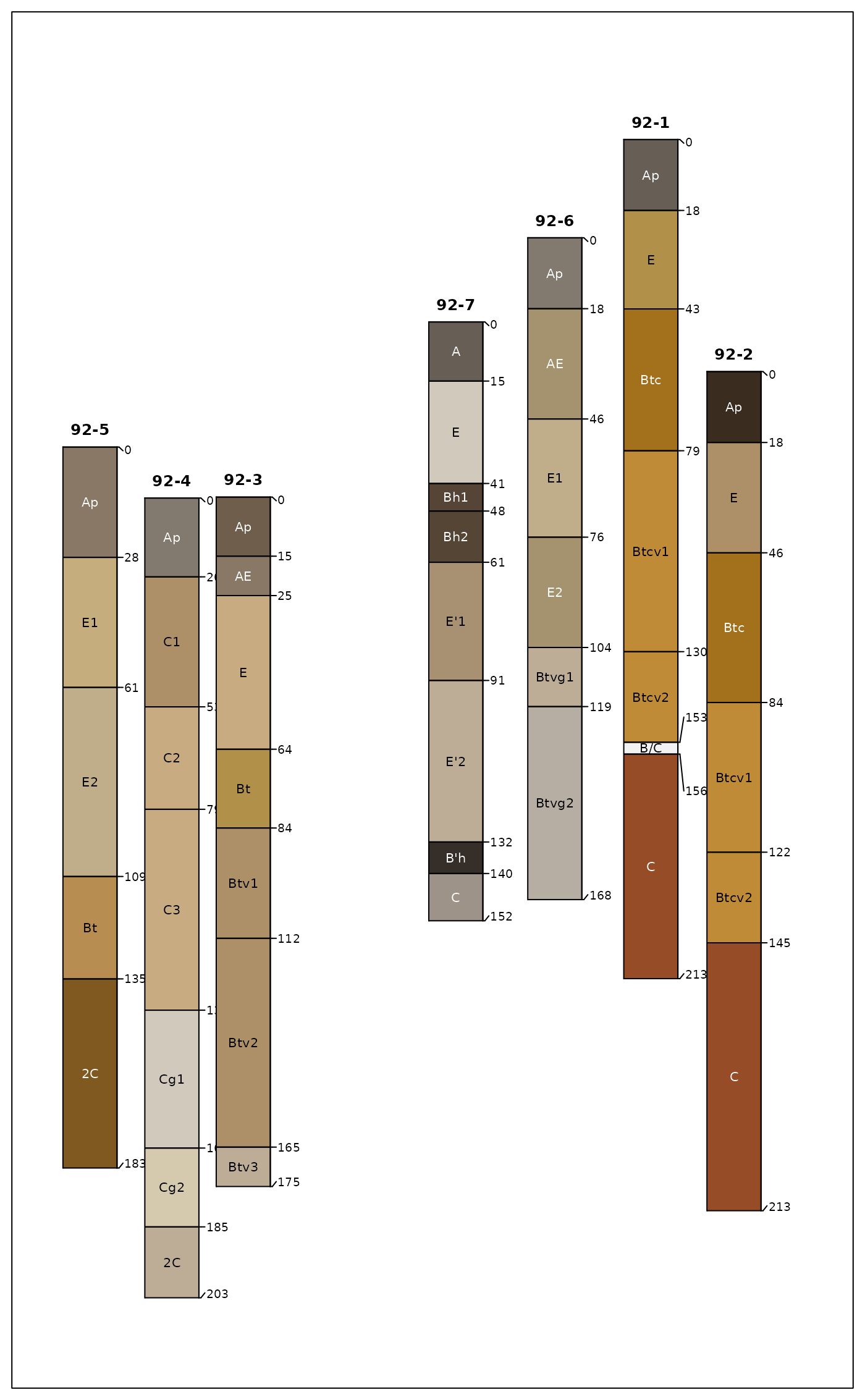Generate a diagram of soil profile sketches from a SoilProfileCollection object. The Introduction to SoilProfileCollection Objects Vignette contains many examples and discussion of the large number of arguments to this function. The Soil Profile Sketches tutorial has longer-form discussion and examples pertaining to suites of related arguments.

Options can be used to conveniently specify sets of arguments that will be used in several calls to plotSPC() within a single R session. For example, arguments can be specified in a named list (.a) and set using: options(.aqp.plotSPC.args = .a). Reset these options via options(.aqp.plotSPC.args = NULL). Arguments explicitly passed to plotSPC() will override arguments set via options().

plotSPC(
x,
color = "soil_color",
width = ifelse(length(x) < 2, 0.15, 0.25),
name = hzdesgnname(x),
name.style = "right-center",
label = idname(x),
raggedBottom = NULL,
hz.depths = FALSE,
hz.depths.offset = ifelse(fixLabelCollisions, 0.03, 0),
hz.depths.lines = fixLabelCollisions,
depth.axis = list(style = "traditional", cex = cex.names * 1.15),
alt.label = NULL,
alt.label.col = "black",
cex.names = 0.5,
cex.id = cex.names + (0.2 * cex.names),
font.id = 2,
srt.id = 0,
print.id = TRUE,
id.style = "auto",
plot.order = 1:length(x),
relative.pos = 1:length(x),
scaling.factor = 1,
y.offset = rep(0, times = length(x)),
x.idx.offset = 0,
n = length(x),
max.depth = ifelse(is.infinite(max(x)), 200, max(x)),
n.depth.ticks = 10,
shrink = FALSE,
shrink.cutoff = 3,
shrink.thin = NULL,
abbr = FALSE,
abbr.cutoff = 5,
divide.hz = TRUE,
hz.distinctness.offset = NULL,
hz.topography.offset = NULL,
hz.boundary.lty = NULL,
density = NULL,
show.legend = TRUE,
col.label = color,
col.palette = c("#5E4FA2", "#3288BD", "#66C2A5", "#ABDDA4", "#E6F598", "#FEE08B",
"#FDAE61", "#F46D43", "#D53E4F", "#9E0142"),
col.palette.bias = 1,
col.legend.cex = 1,
n.legend = 8,
lwd = 1,
lty = 1,
default.color = grey(0.95),
fixLabelCollisions = hz.depths,
fixOverlapArgs = list(method = "E", q = 1),
cex.depth.axis = cex.names,
axis.line.offset = -2,
plot.depth.axis = TRUE,
...
)

# S4 method for SoilProfileCollection,ANY
plot(x, y, ...)

## Arguments

x

a SoilProfileCollection object

color

quoted column name containing R-compatible color descriptions, or numeric / categorical data to be displayed thematically; see details

width

scaling of profile widths (typically 0.1 - 0.4)

name

quoted column name of the (horizon-level) attribute containing horizon designations or labels, if missing hzdesgnname(x) is used. Suppress horizon name printing by setting name = NA or name = ''.

name.style

one of several possible horizon designations labeling styles: c('right-center', 'left-center', 'left-top', 'center-center', 'center-top')

label

quoted column name of the (site-level) attribute used to identify profile sketches

raggedBottom

either quoted column name of the (site-level) attribute (logical) used to mark profiles with a truncated lower boundary, or FALSE suppress ragged bottom depths when max.depth < max(x)

hz.depths

logical, annotate horizon top depths to the right of each sketch (FALSE)

hz.depths.offset

numeric, user coordinates for left-right adjustment for horizon depth annotation; reasonable values are usually within 0.01-0.05 (default: 0)

hz.depths.lines

logical, draw segments between horizon depth labels and actual horizon depth; this is useful when including horizon boundary distinctness and/or fixLabelCollisions = TRUE

depth.axis

logical or list. Use a logical to suppress (FALSE) or add depth axis using defaults (TRUE). Use a list to specify one or more of:

• style: character, one of 'traditional', 'compact', or 'tape'

• line: numeric, negative values move axis to the left (does not apply to style = 'tape')

• cex: numeric, scaling applied to entire depth axis

• interval: numeric, axis interval See examples.

alt.label

quoted column name of the (site-level) attribute used for secondary annotation

alt.label.col

color used for secondary annotation text

cex.names

baseline character scaling applied to all text labels

cex.id

character scaling applied to label

font.id

font style applied to label, default is 2 (bold)

srt.id

rotation applied to label, only when id.style = 'top'

print.id

logical, print label above/beside each profile? (TRUE)

id.style

label printing style: 'auto' (default) = simple heuristic used to select from: 'top' = centered above each profile, 'side' = 'along the top-left edge of profiles'

plot.order

integer vector describing the order in which individual soil profiles should be plotted

relative.pos

vector of relative positions along the x-axis, within {1, n}, ignores plot.order see details

logical, add to an existing figure

scaling.factor

vertical scaling of profile depths, useful for adding profiles to an existing figure

y.offset

numeric vector of vertical offset for top of profiles in depth units of x, can either be a single numeric value or vector of length = length(x). A vector of y-offsets will be automatically re-ordered according to plot.order.

x.idx.offset

integer specifying horizontal offset from 0 (left-hand edge)

n

integer describing amount of space along x-axis to allocate, defaults to length(x)

max.depth

numeric. The lower depth for all sketches, deeper profiles are truncated at this depth. Use larger values to arbitrarily extend the vertical dimension, convenient for leaving extract space for annotation.

n.depth.ticks

suggested number of ticks in depth scale

shrink

logical, reduce character scaling for 'long' horizon by 80%

shrink.cutoff

character length defining 'long' horizon names

shrink.thin

integer, horizon thickness threshold for shrinking horizon names by 80%, only activated when shrink = TRUE (NULL = no shrinkage)

abbr

logical, abbreviate label

abbr.cutoff

suggested minimum length for abbreviated label

divide.hz

logical, divide horizons with line segment? (TRUE), see details

hz.distinctness.offset

NULL, or quoted column name (horizon-level attribute) containing vertical offsets used to depict horizon boundary distinctness (same units as profiles), see details and hzDistinctnessCodeToOffset(); consider setting hz.depths.lines = TRUE when used in conjunction with hz.depths = TRUE

hz.topography.offset

NULL, or quoted column name (horizon-level attribute) containing offsets used to depict horizon boundary topography (same units as profiles), see details and hzTopographyCodeToOffset()

hz.boundary.lty

quoted column name (horizon-level attribute) containing line style (integers) used to encode horizon topography

density

fill density used for horizon color shading, either a single integer or a quoted column name (horizon-level attribute) containing integer values (default is NULL, no shading)

show.legend

logical, show legend? (default is TRUE)

col.label

thematic legend title

col.palette

color palette used for thematic sketches (default is rev(brewer.pal(10, 'Spectral')))

col.palette.bias

color ramp bias (skew), see colorRamp()

col.legend.cex

scaling of thematic legend

n.legend

approximate number of classes used in numeric legend, max number of items per row in categorical legend

lwd

line width multiplier used for sketches

lty

line style used for sketches

default.color

default horizon fill color used when color attribute is NA

fixLabelCollisions

use fixOverlap() to attempt fixing hz depth labeling collisions, will slow plotting of large collections; enabling also sets hz.depths.lines = TRUE. Additional arguments to fixOverlap() can be passed via fixOverlapArgs. Overlap collisions cannot be fixed within profiles containing degenerate or missing horizon depths (e.g. top == bottom).

fixOverlapArgs

a named list of arguments to fixOverlap(). Overlap adjustments are attempted using electrostatic simulation with arguments: list(method = 'E', q = 1). Alternatively, select adjustment by simulated annealing via list(method = 'S'). See electroStatics_1D() and SANN_1D() for details.

cex.depth.axis

(deprecated, use depth.axis instead) character scaling applied to depth scale

axis.line.offset

(deprecated, use depth.axis instead) horizontal offset applied to depth axis (default is -2, larger numbers move the axis to the right)

plot.depth.axis

(deprecated, use depth.axis instead) logical, plot depth axis?

...

other arguments passed into lower level plotting functions

y

(not used)

## Details

Depth limits (max.depth) and number of depth ticks (n.depth.ticks) are suggestions to the pretty() function. You may have to tinker with both parameters to get what you want.

The 'side' id.style is useful when plotting a large collection of profiles, and/or, when profile IDs are long.

If the column containing horizon designations is not specified (the name argument), a column (presumed to contain horizon designation labels) is guessed based on regular expression matching of the pattern 'name'--this usually works, but it is best to manual specify the name of the column containing horizon designations.

The color argument can either name a column containing R-compatible colors, possibly created via munsell2rgb(), or column containing either numeric or categorical (either factor or character) values. In the second case, values are converted into colors and displayed along with a simple legend above the plot. Note that this functionality makes several assumptions about plot geometry and is most useful in an interactive setting.

Adjustments to the legend can be specified via col.label (legend title), col.palette (palette of colors, automatically expanded), col.legend.cex (legend scaling), and n.legend (approximate number of classes for numeric variables, or, maximum number of legend items per row for categorical variables). Currently, plotSPC will only generate two rows of legend items. Consider reducing the number of classes if two rows isn't enough room.

Profile sketches can be added according to relative positions along the x-axis (vs. integer sequence) via relative.pos argument. This should be a vector of positions within {1,n} that are used for horizontal placement. Default values are 1:length(x). Care must be taken when both plot.order and relative.pos are used simultaneously: relative.pos specifies horizontal placement after sorting. addDiagnosticBracket() and addVolumeFraction() use the relative.pos values for subsequent annotation.

Relative positions that are too close will result in overplotting of sketches. Adjustments to relative positions such that overlap is minimized can be performed with fixOverlap(pos), where pos is the original vector of relative positions.

The x.idx.offset argument can be used to shift a collection of pedons from left to right in the figure. This can be useful when plotting several different SoilProfileCollection objects within the same figure. Space must be pre-allocated in the first plotting call, with an offset specified in the second call. See examples below.

Horizon depths (e.g. cm) are converted to figure y-coordinates via: y = (depth * scaling.factor) + y.offset.

## Note

A new plot of soil profiles is generated, or optionally added to an existing plot.

fixOverlap(), explainPlotSPC(), SoilProfileCollection, pretty(), hzDistinctnessCodeToOffset(), addBracket(), profileGroupLabels()

D.E. Beaudette

## Examples


# keep examples from using more than 2 cores

# example data
data(sp1)
# usually best to adjust margins
par(mar = c(0,0,3,0))

sp1$soil_color <- with(sp1, munsell2rgb(hue, value, chroma)) # promote to SoilProfileCollection depths(sp1) <- id ~ top + bottom # init horizon designation hzdesgnname(sp1) <- 'name' # plot profiles plotSPC(sp1, id.style = 'side') # title, note line argument: title('Sample Data 1', line = 1, cex.main = 0.75)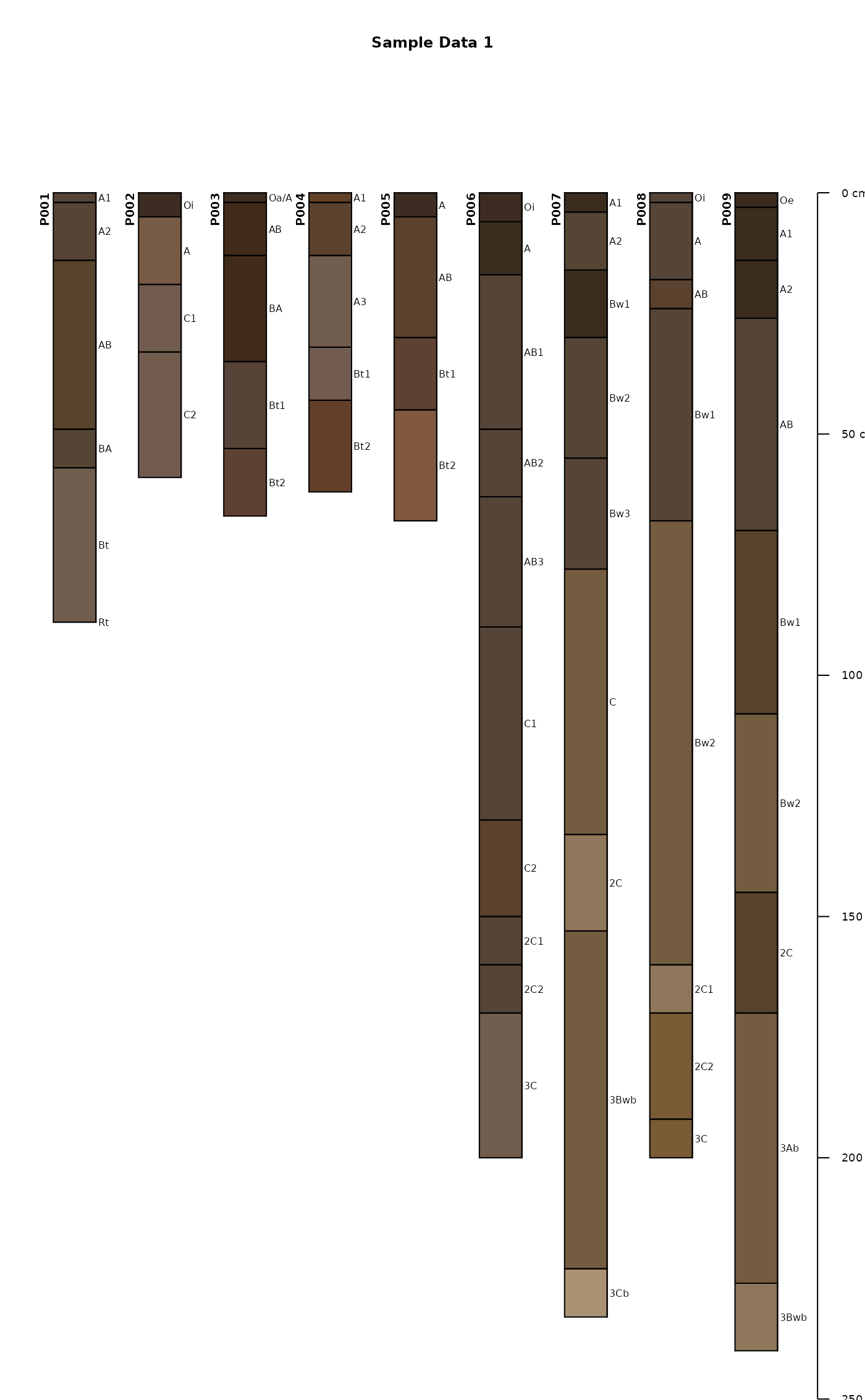# plot profiles without horizon-line divisions plotSPC(sp1, divide.hz = FALSE)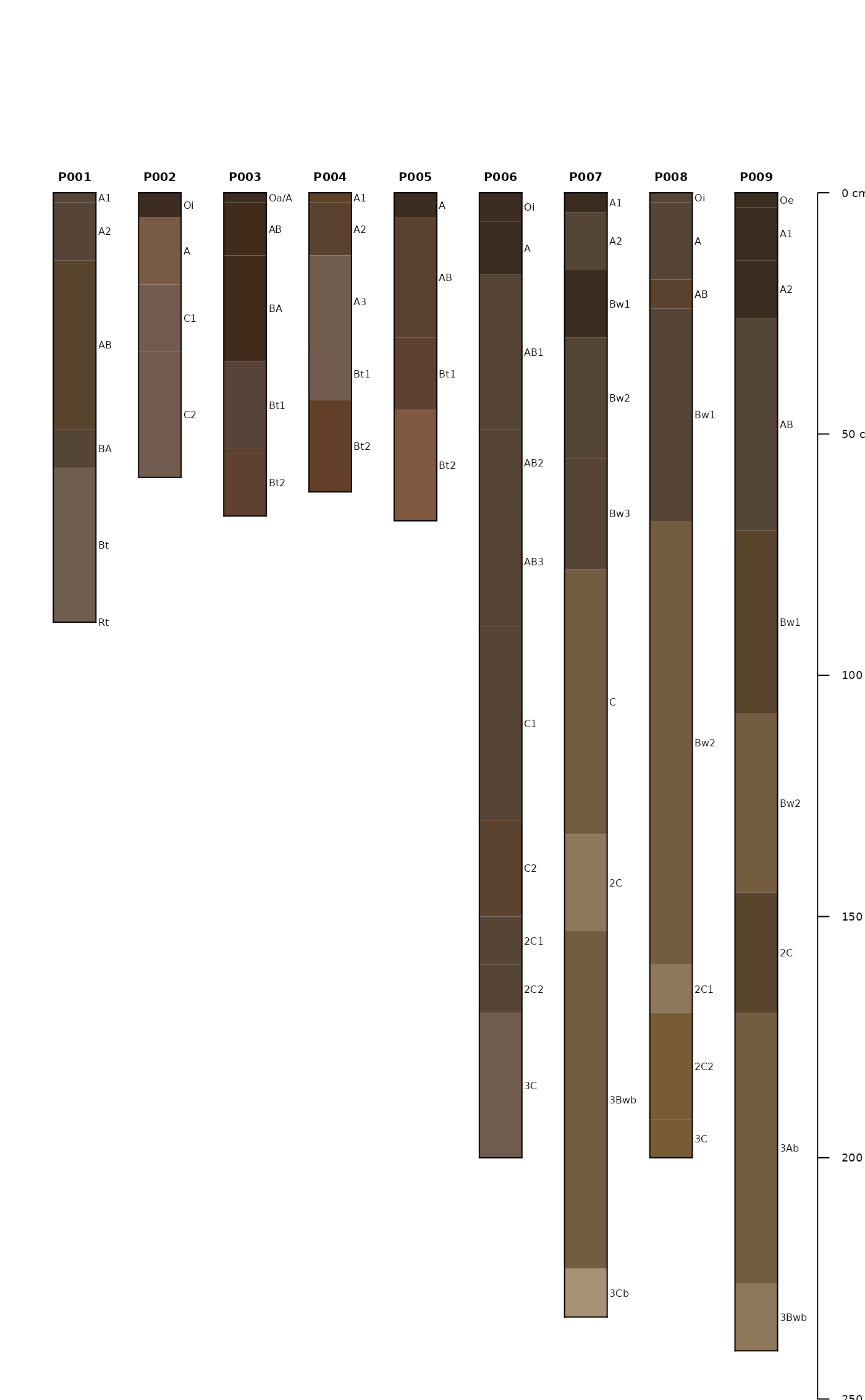# diagonal lines encode horizon boundary distinctness sp1$hzD <- hzDistinctnessCodeToOffset(sp1$bound_distinct) plotSPC(sp1, hz.distinctness.offset = 'hzD', name.style = 'center-center')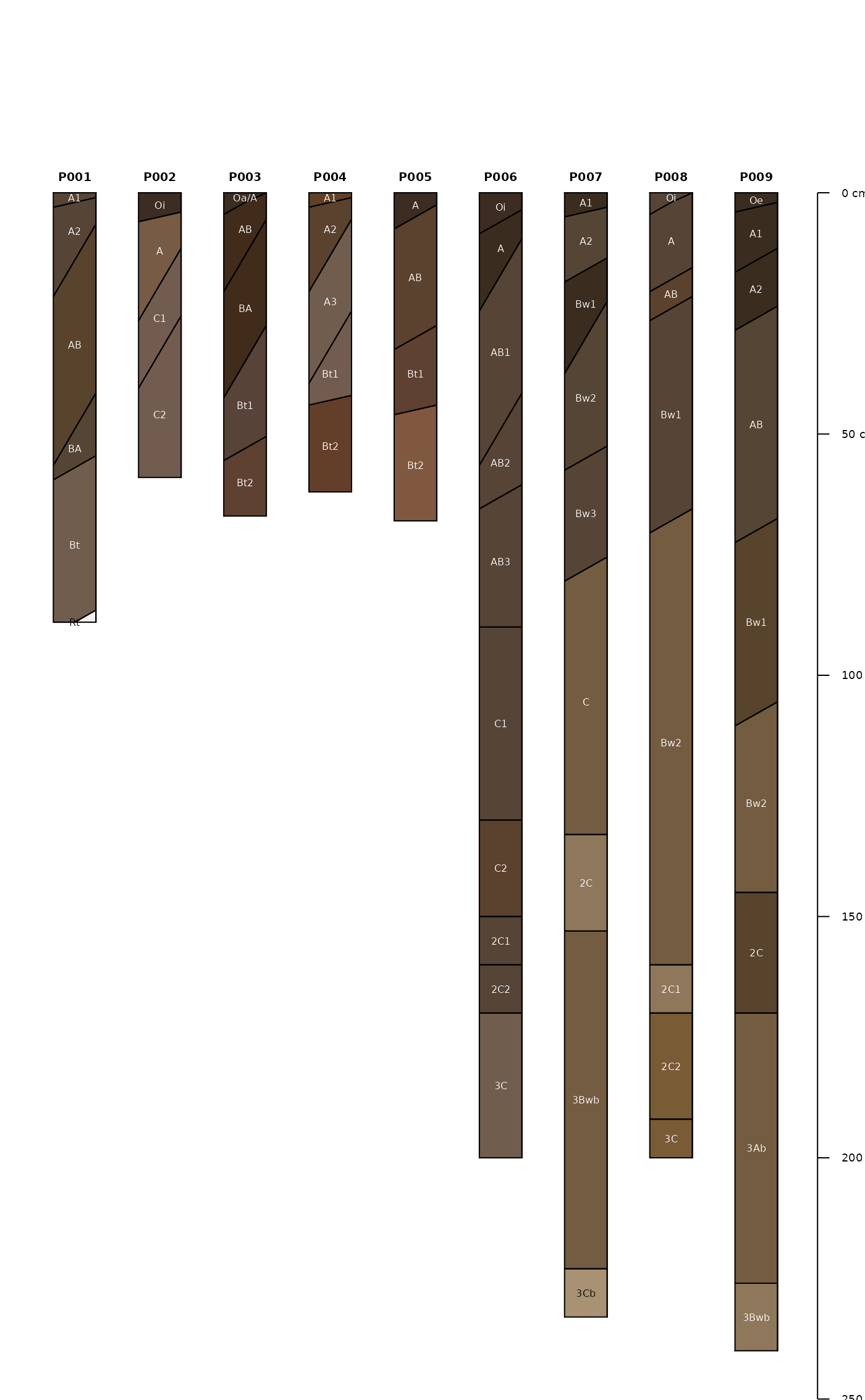# plot horizon color according to some property data(sp4) depths(sp4) <- id ~ top + bottom hzdesgnname(sp4) <- 'name' plotSPC(sp4, color = 'clay')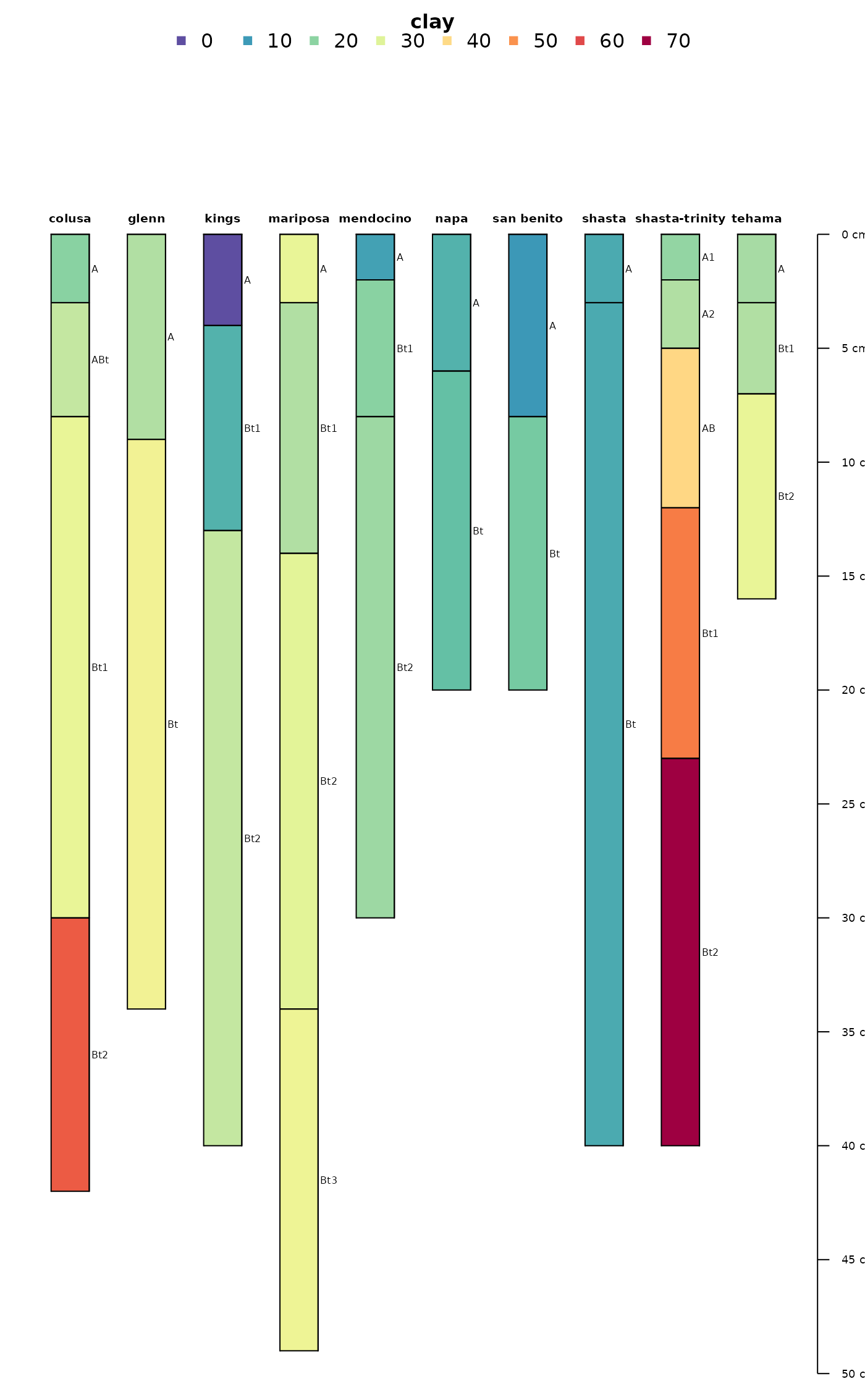# another example data(sp2) depths(sp2) <- id ~ top + bottom hzdesgnname(sp2) <- 'name' site(sp2) <- ~ surface # some of these profiles are very deep, truncate plot at 400cm # label / re-order with site-level attribute: surface plotSPC(sp2, label = 'surface', plot.order = order(sp2$surface),
max.depth = 400)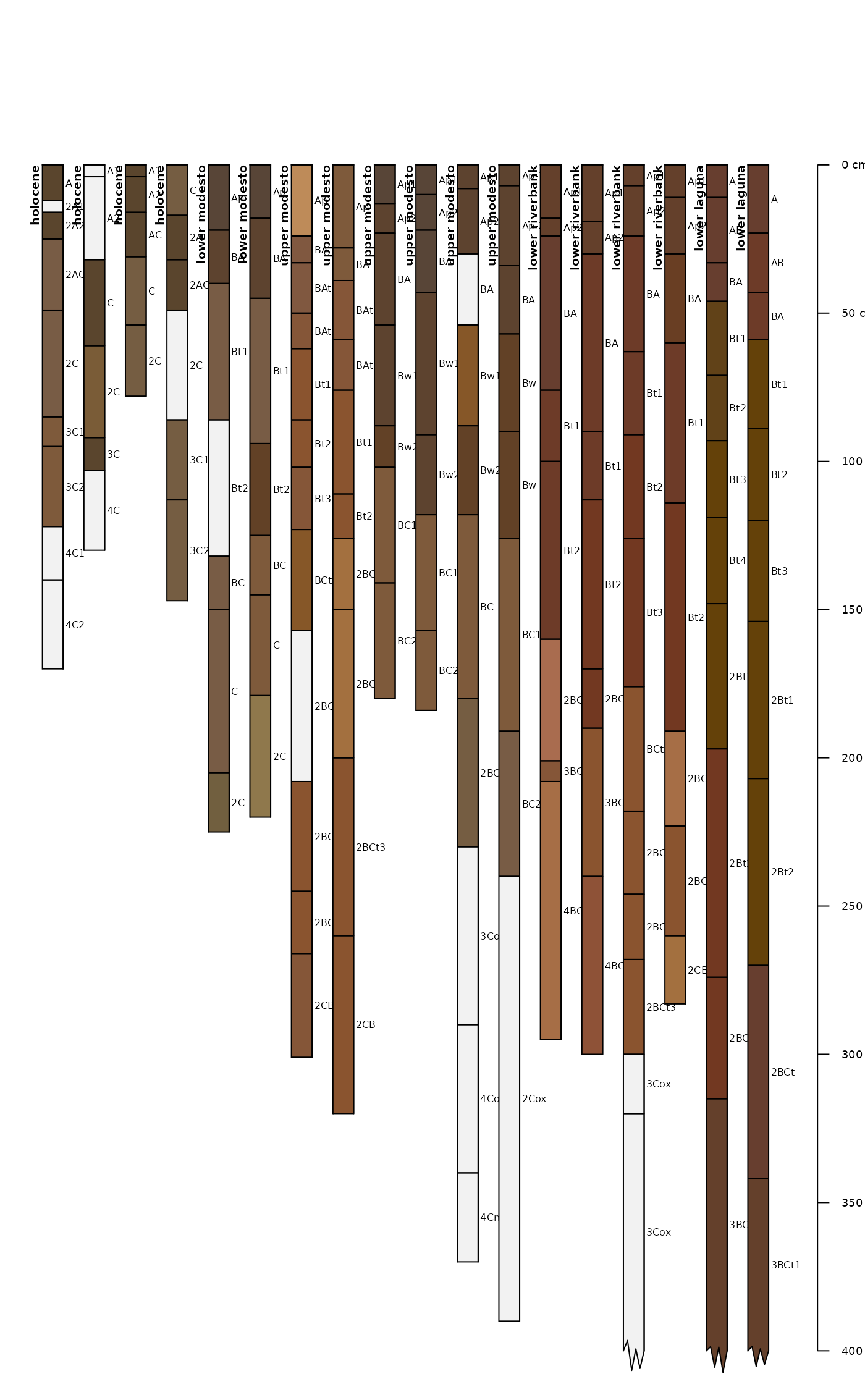# example using a categorical attribute
plotSPC(sp2, color = "plasticity",
max.depth = 400)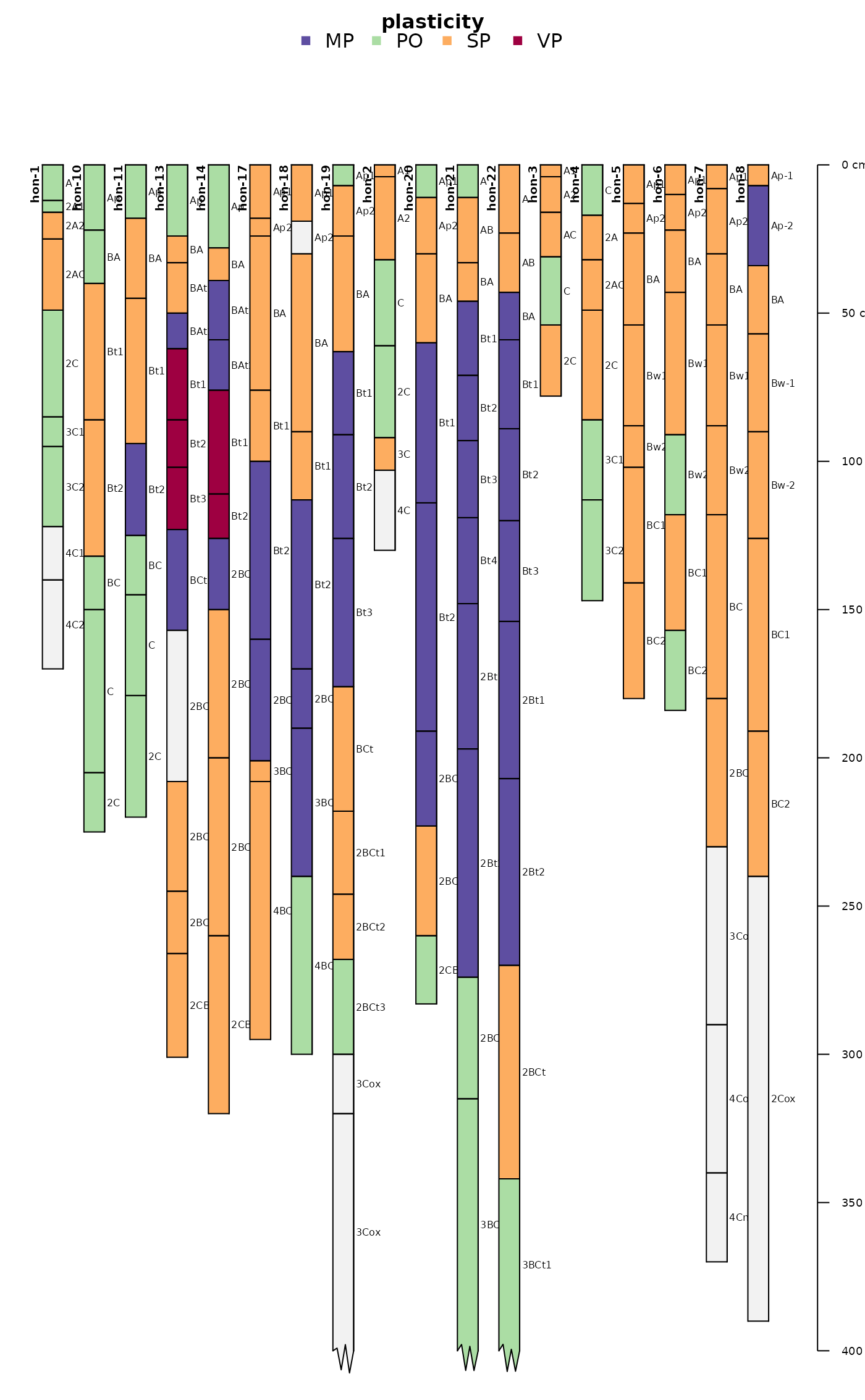# plot two SPC objects in the same figure
par(mar = c(1,1,1,1))

# plot the first SPC object and
# allocate space for the second SPC object
plotSPC(sp1, n = length(sp1) + length(sp2))

# plot the second SPC, starting from the first empty space
plotSPC(sp2, x.idx.offset = length(sp1), add = TRUE)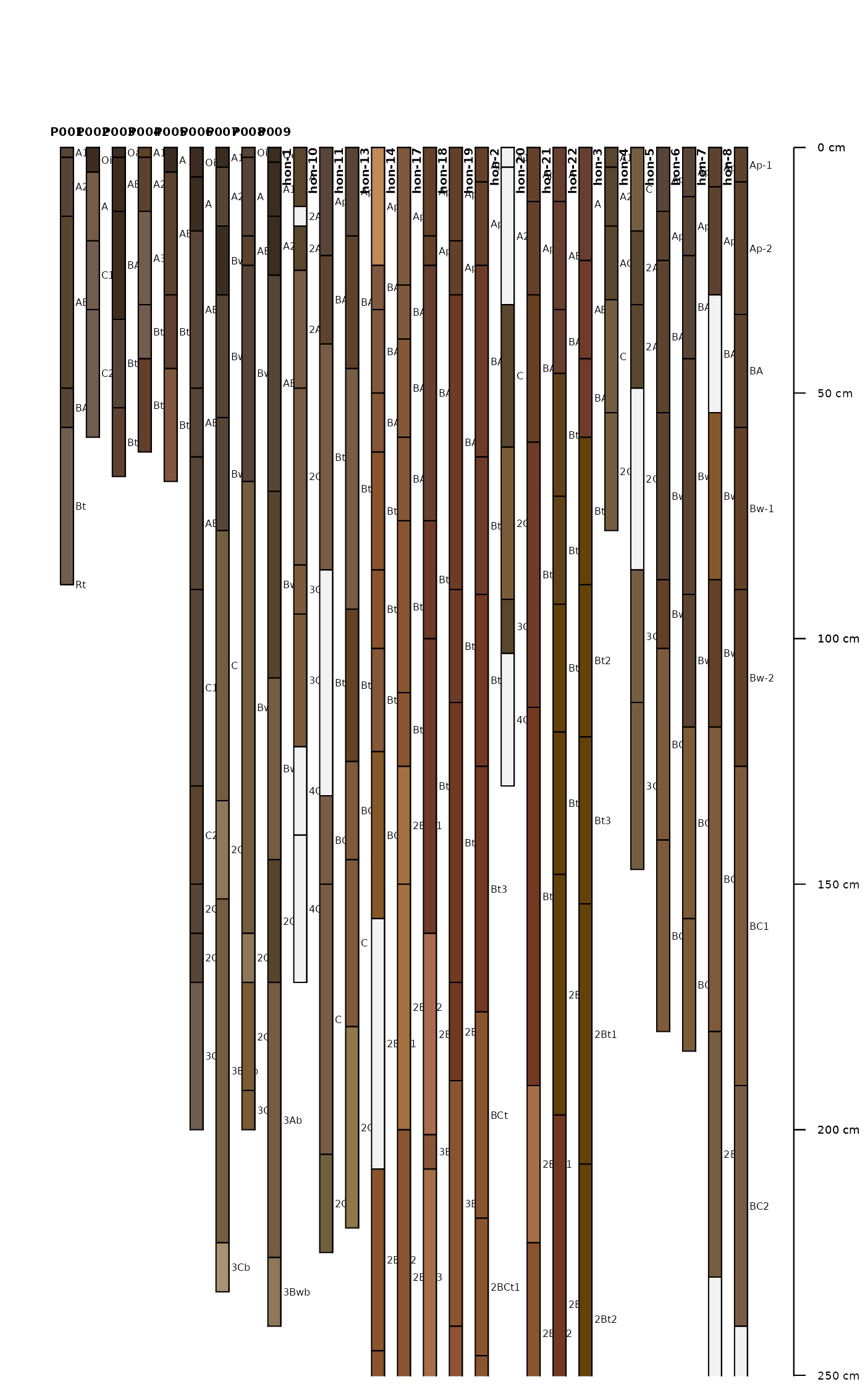##
## demonstrate horizon designation shrinkage
##

data("jacobs2000")

# shrink "long" horizon names
plotSPC(
jacobs2000,
name = 'name',
name.style = 'center-center',
shrink = TRUE,
cex.names = 0.8
)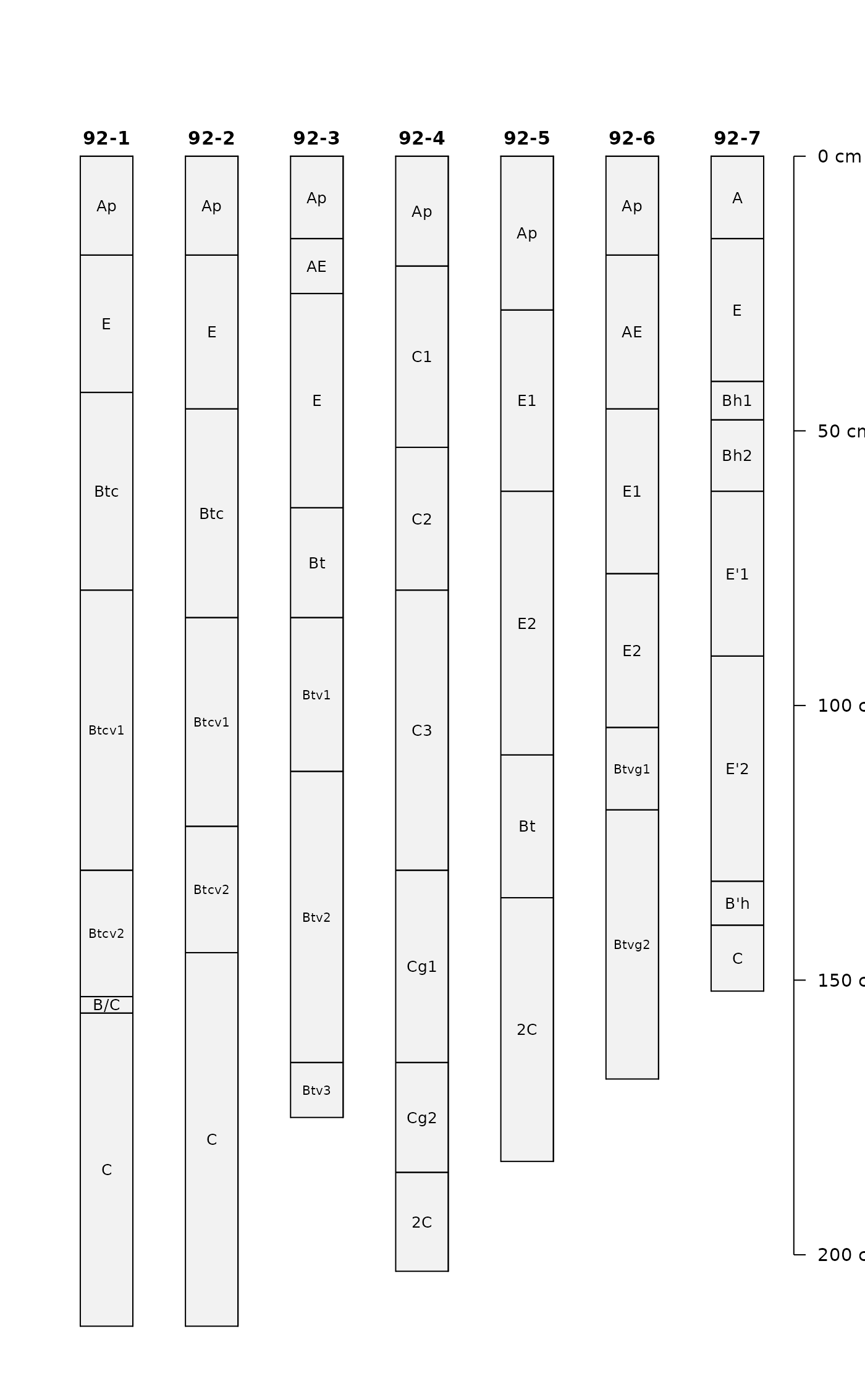# shrink horizon names in "thin" horizons
plotSPC(
jacobs2000,
name = 'name',
name.style = 'center-center',
shrink = TRUE,
shrink.thin = 15,
cex.names = 0.8,
)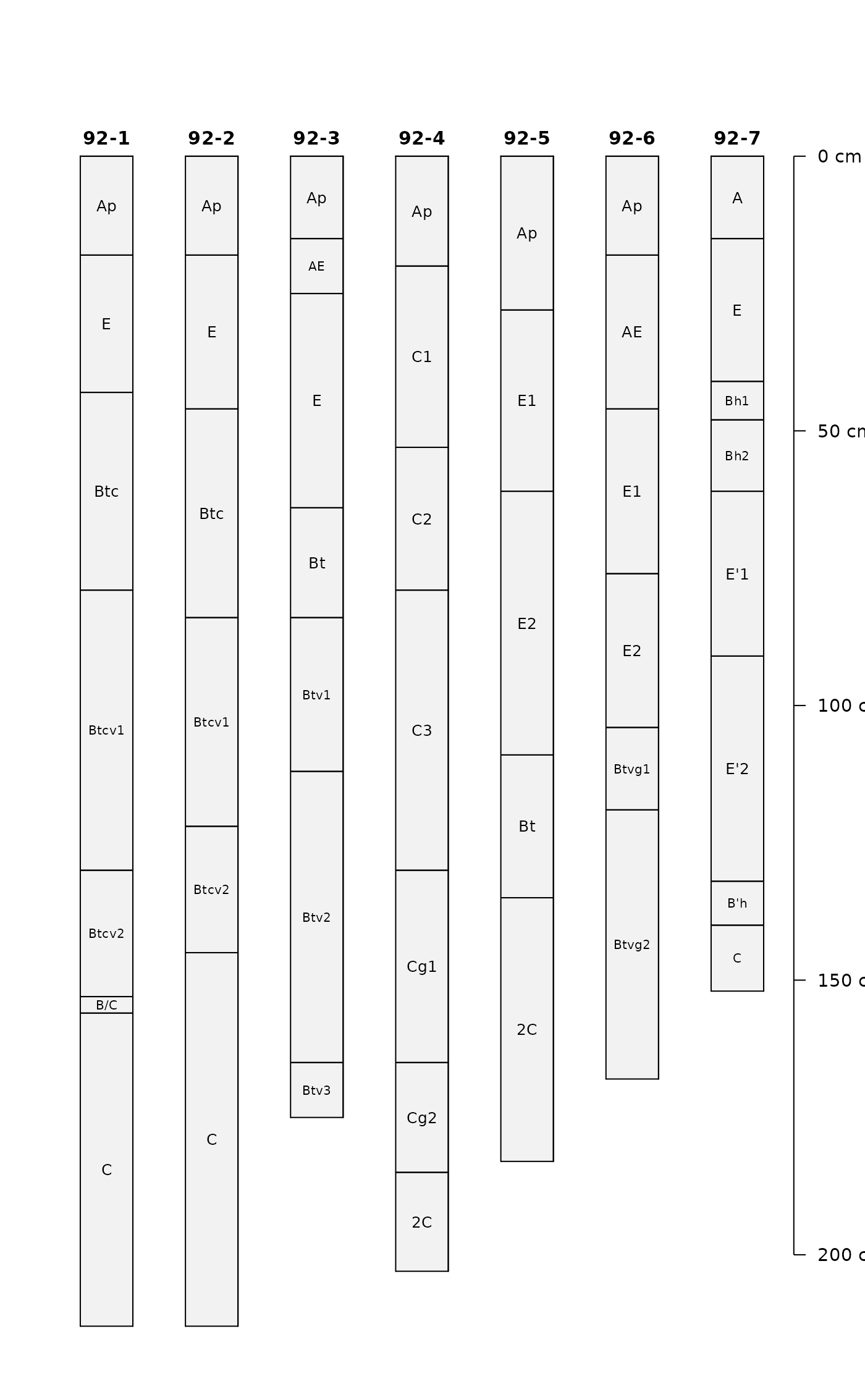##
##

data(sp3)
depths(sp3) <- id ~ top + bottom

# make some fake categorical data
horizons(sp3)fake.data <- sample(letters[1:15], size = nrow(sp3), replace=TRUE) # better margins par(mar=c(0,0,3,1)) # note that there are enough colors for 15 classes (vs. previous limit of 10) # note that the legend is split into 2 rows when length(classes) > n.legend argument plotSPC(sp3, color='fake.data', name='fake.data', cex.names=0.8)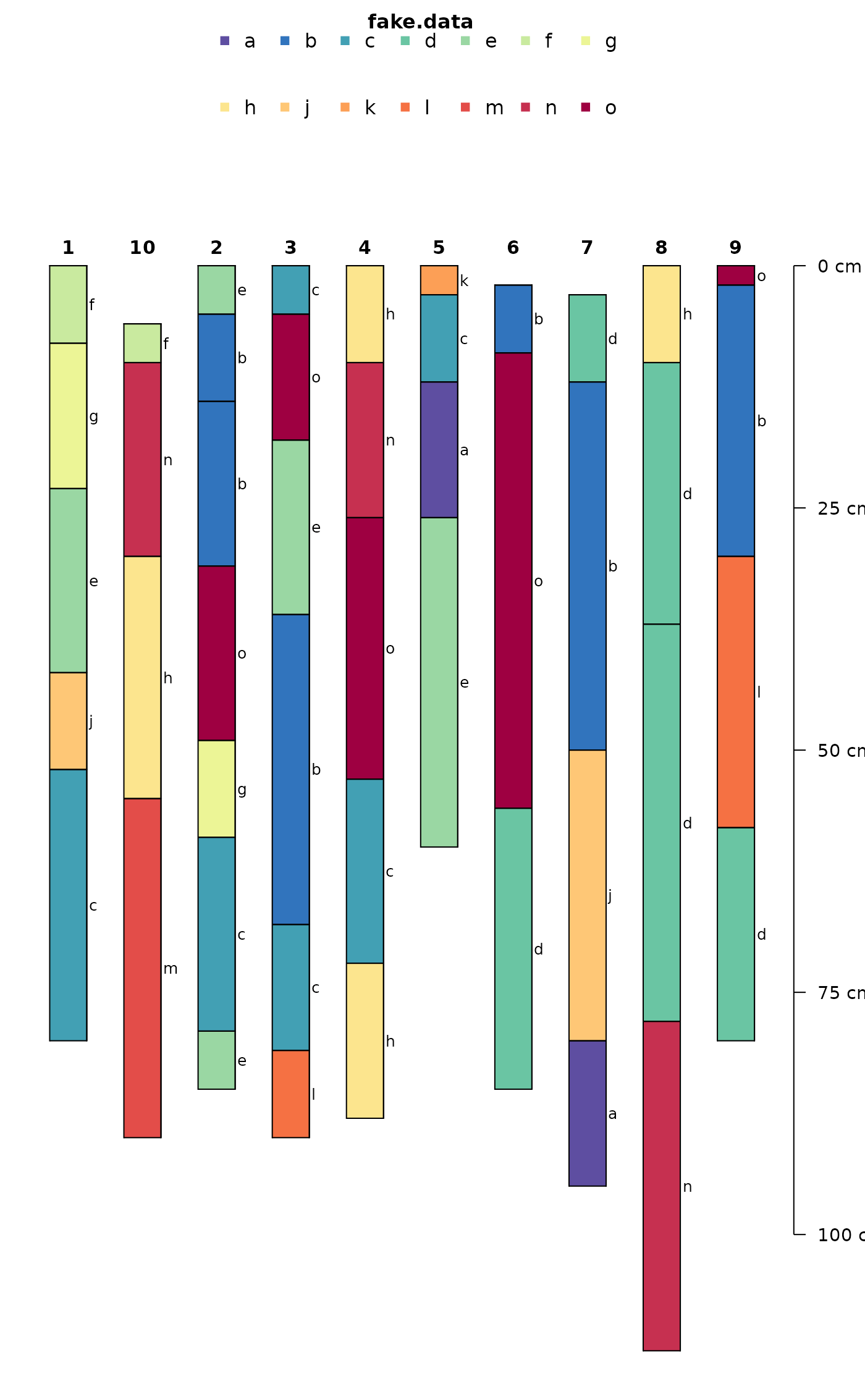# make enough room in a single legend row plotSPC(sp3, color='fake.data', name='fake.data', cex.names=0.8, n.legend=15)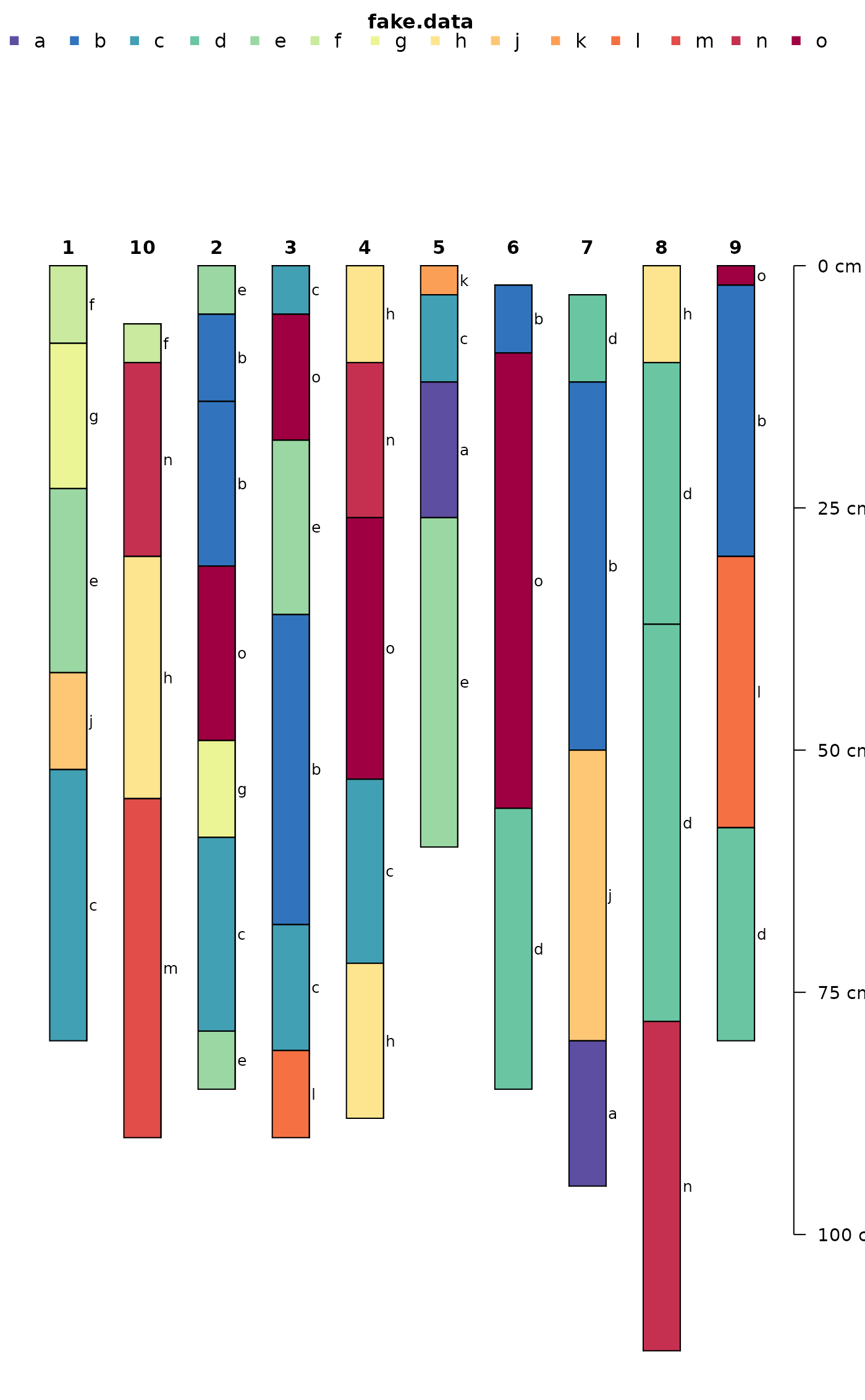## ## demonstrate y.offset argument ## must be of length 1 or length(x) ## # example data and local copy data("jacobs2000") x <- jacobs2000 hzdesgnname(x) <- 'name' # y-axis offsets, simulating a elevation along a hillslope sequence # same units as horizon depths in x # same order as profiles in x y.offset <- c(-5, -10, 22, 65, 35, 15, 12) par(mar = c(0, 0, 2, 2)) # y-offset at 0 plotSPC(x, color = 'matrix_color', cex.names = 0.66)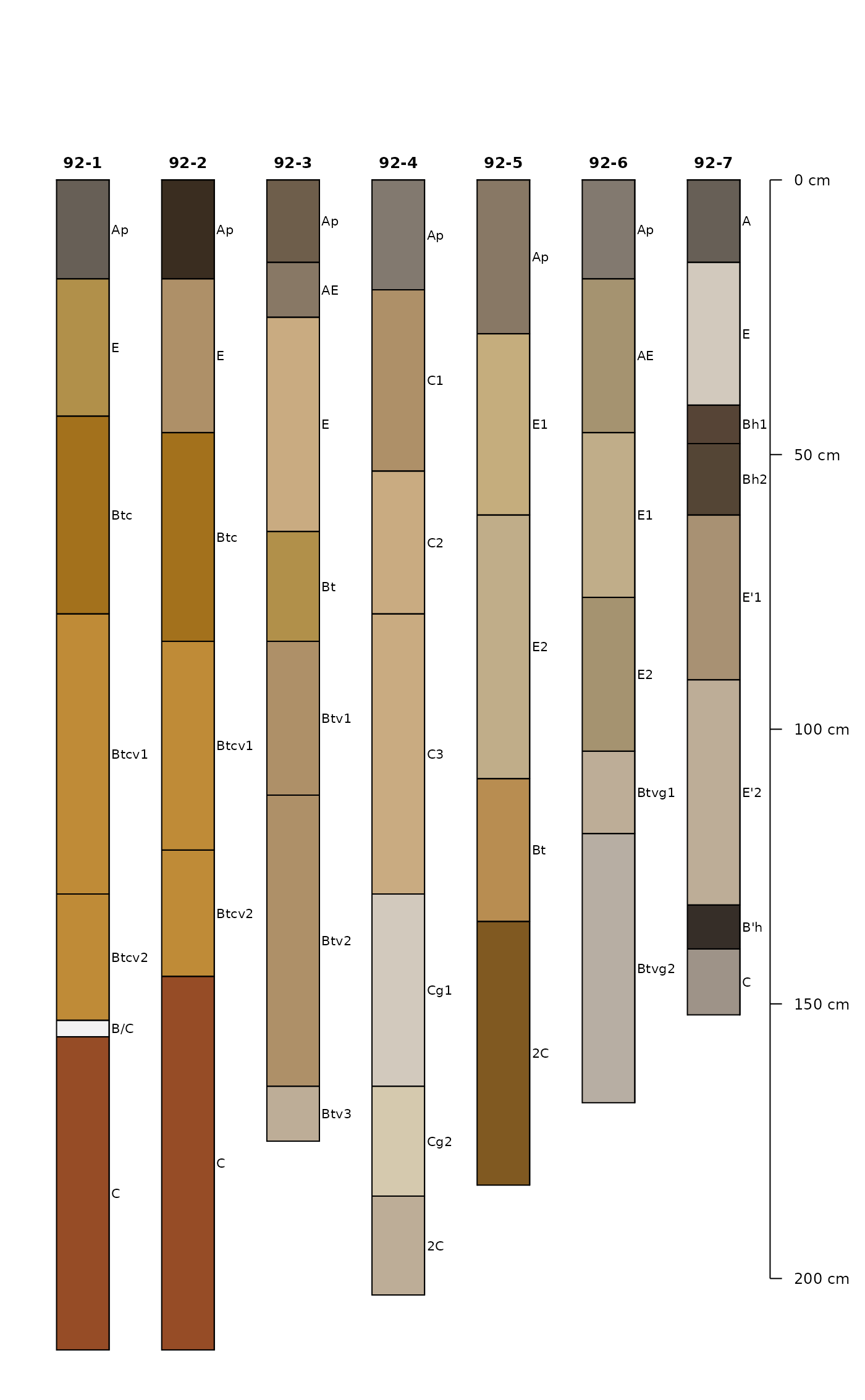# constant adjustment to y-offset plotSPC(x, color = 'matrix_color', cex.names = 0.66, y.offset = 50)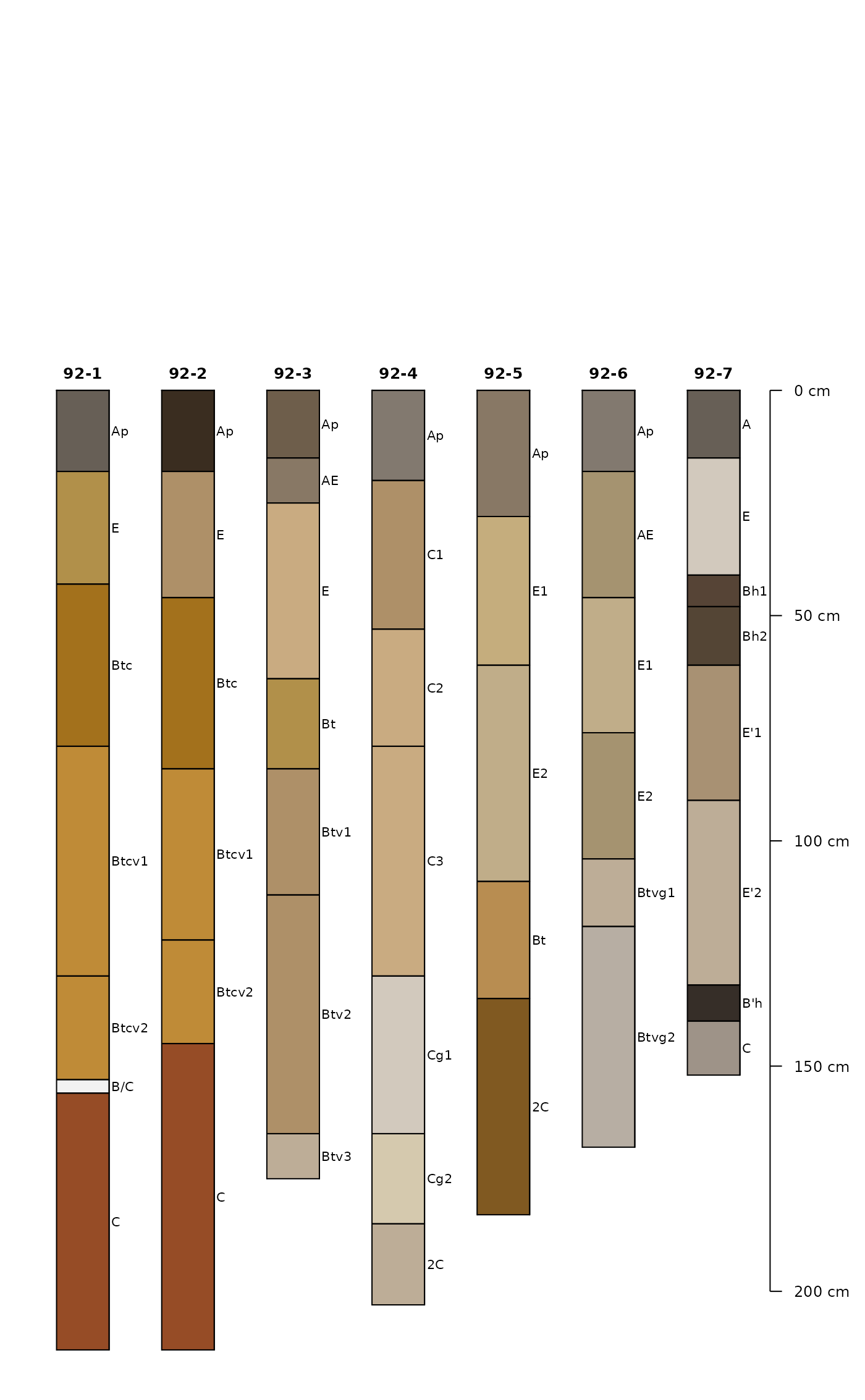# attempt using invalid y.offset # warning issued and default value of '0' used # plotSPC(x, color = 'matrix_color', cex.names = 0.66, y.offset = 1:2) # variable y-offset # fix overlapping horizon depth labels par(mar = c(0, 0, 1, 0)) plotSPC( x, y.offset = y.offset, color = 'matrix_color', cex.names = 0.75, shrink = TRUE, hz.depths = TRUE, hz.depths.offset = 0.05, fixLabelCollisions = TRUE, name.style = 'center-center' ) #> depth axis is disabled when more than 1 unique y offsets are supplied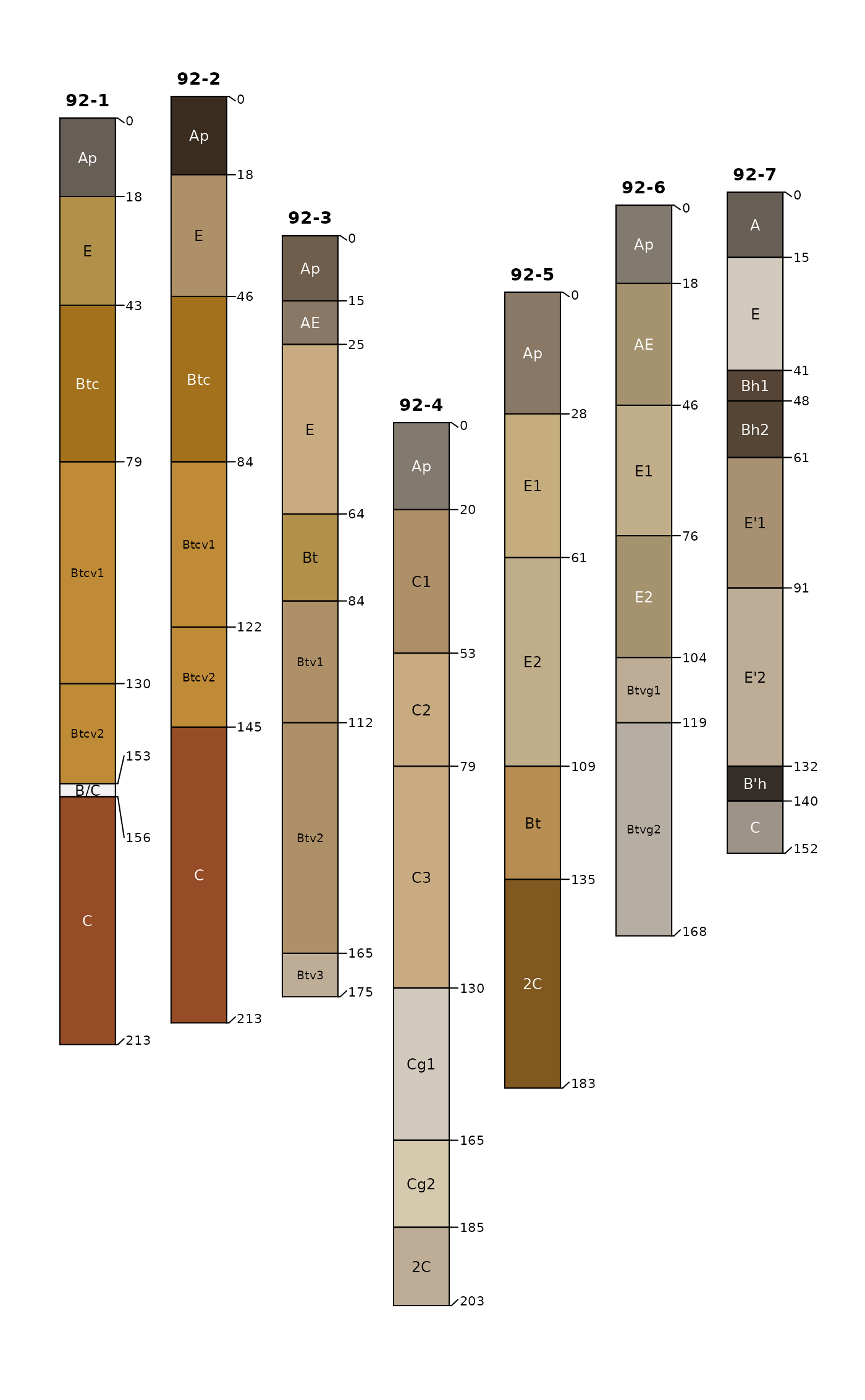# random y-axis offsets yoff <- runif(n = length(x), min = 1, max = 100) # random gradient of x-positions xoff <- runif(n = length(x), min = 1, max = length(x)) # note profiles overlap plotSPC(x, relative.pos = xoff, y.offset = yoff, color = 'matrix_color', cex.names = 0.66, hz.depths = TRUE, name.style = 'center-center' ) #> depth axis is disabled when more than 1 unique y offsets are supplied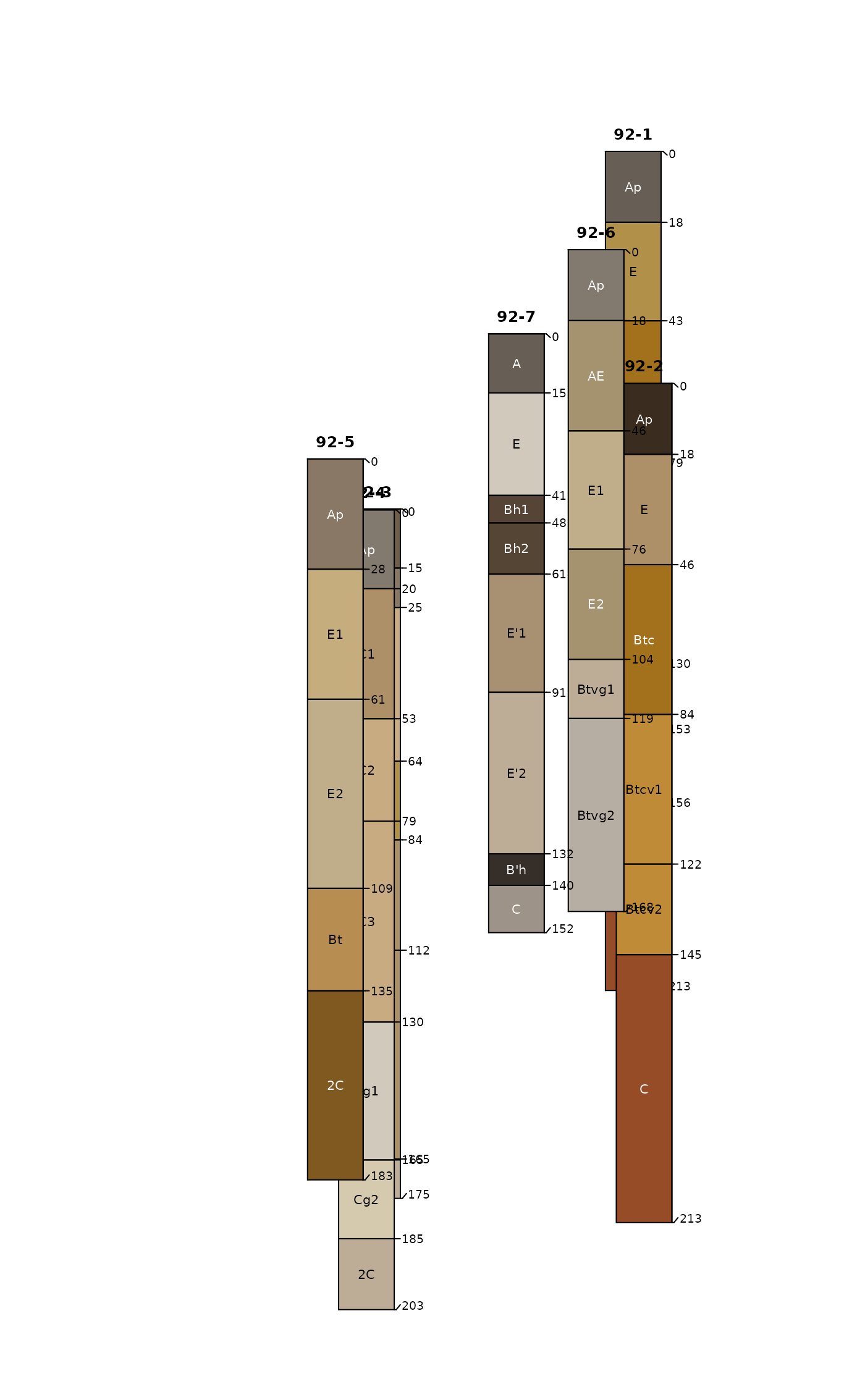# align / adjust relative x positions set.seed(111) pos <- alignTransect(xoff, x.min = 1, x.max = length(x), thresh = 0.65) #> 162 iterations # y-offset is automatically re-ordered according to # plot.order par(mar = c(0.5, 0.5, 0.5, 0.5)) plotSPC(x, plot.order = posorder,
relative.pos = pos\$relative.pos,
y.offset = yoff,
color = 'matrix_color',
cex.names = 0.66,
hz.depths = TRUE,
name.style = 'center-center'
)
#> depth axis is disabled when more than 1 unique y offsets are supplied

box()